# Software Engineering | Application Composition Estimation Model (COCOMO II | Stage 1)

Application Composition Estimation Model allows one to estimate the cost, effort at the stage 1 of the COCOMO II Model.

In this model size is first estimated using Object Points. Object Points are easy to identify and count. Object Points defines screen, reports, third generation (3GL) modules as objects.

Object Point estimation is a new size estimation technique but it is well suited in Application Composition Sector.

Estimation of Efforts:
Following steps are taken to estimate effort to develop a project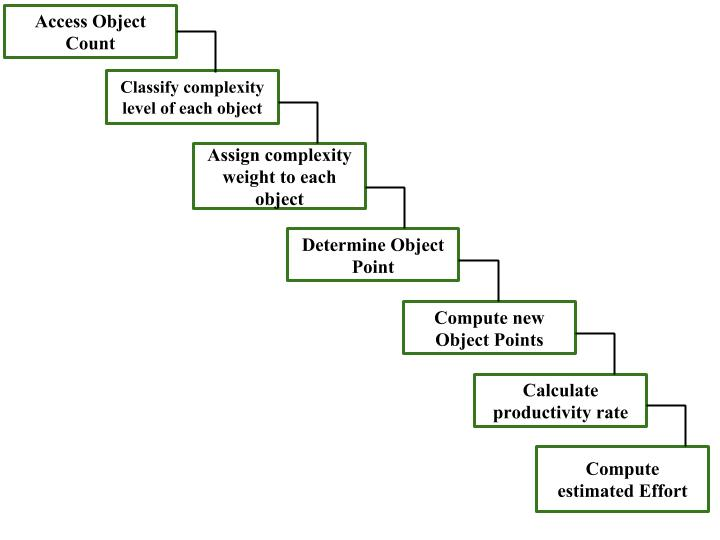Step-1: Access Object counts
Estimate the number of screens, reports and 3GL components that will comprise this application.

Step-2: Classify complexity levels of each object
We have to classify each object instance into simple, medium and difficult complexity level depending on values of its characteristics.
Complexity levels are assigned according to the given table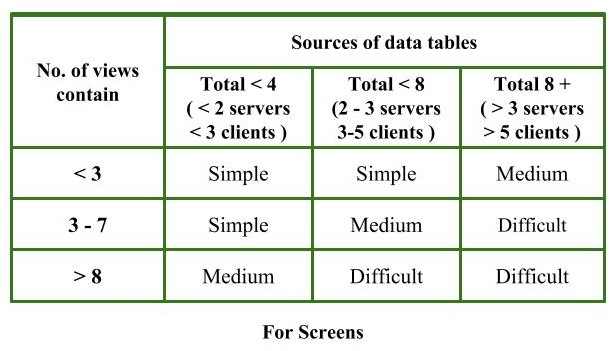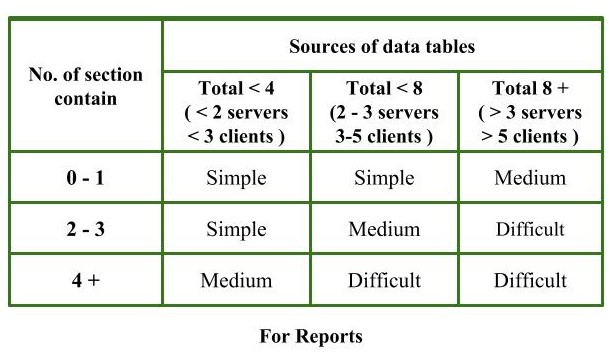Step-3: Assign complexity weights to each object
The weights are used for three object types i.e, screens, reports and 3GL components.
Complexity weight are assigned according to object’s complexity level using following table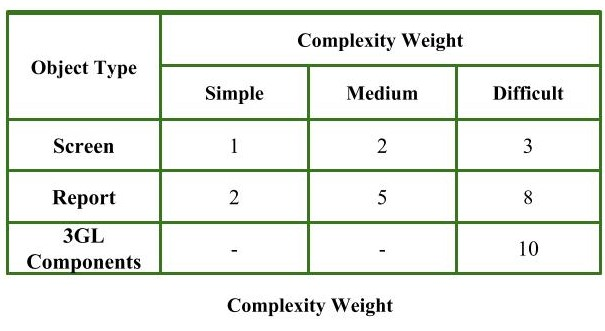Step-4: Determine Object Points
Add all the weighted object instances to get one number and this is known as object point count.

```Object Point
= Sigma (number of object instances)
* (Complexity weight of each object instance)
```

Step-5: Compute New Object Points (NOP)
We have to estimate the %reuse to be achieved in a project.
Depending on %reuse

```NOP = [(object points) * (100 - %reuse)]/100
```

NOP are the object point that will need to be developed and differ from the object point count because there may be reuse of some object instance in project.

Step-6: Calculate Productivity rate (PROD)
Productivity rate is calculated on the basis of information given about developer’s experience and capability.
For calculating it, we use following table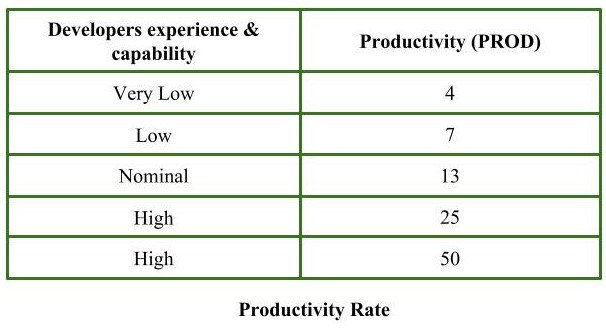Step-7: Compute the estimated Effort
Effort to develop a project can be calculated as

```Effort = NOP/PROD
```

Effort is measured in person-month.

Example:
Consider a database application project with

1. The application has four screens with four views each and seven data tables for three servers and four clients.
2. Application may generate two reports of six section each from seven data tables for two servers and three clients.

10% reuse of object points.
Developer’s experience and capability in similar environment is low. Calculate the object point count, New object point and effort to develop such project.

Step-1:
Number of screens = 4
Number of records = 2

Step-2:
For screens,
Number of views = 4
Number of data tables = 7
Number of servers = 3
Number of clients = 4
by using above given information and table (For Screens),
Complexity level for each screen = medium

For reports,
Number of sections = 6
Number of data tables = 7
Number of servers = 2
Number of clients = 3
by using above given information and table (For Reports),
Complexity level for each report = difficult

Step-3:
By using complexity weight table we can assign complexity weight to each object instance depending upon their complexity level.
Complexity weight for each screen = 2
Complexity weight for each report = 8

Step-4:

```Object point count
= sigma (Number of object instances) * (its Complexity weight)
= 4 * 2 + 2 * 8 = 24 ```

Step-5:

```%reuse of object points = 10% (given)
NOP = [object points * (100 - %reuse)]/100
= [24 * (100 -10)]/100 = 21.6 ```

Step-6:
Developer’s experience and capability is low (given)
Using information given about developer and productivity rate table
Productivity rate (PROD) of given project = 7

Step-7:

```Effort
= NOP/PROD
= 21.6/7
= 3.086 person-month ```

Therefore, effort to develop the given project = 3.086 person-month.

Attention reader! Don’t stop learning now. Get hold of all the important CS Theory concepts for SDE interviews with the CS Theory Course at a student-friendly price and become industry ready.

My Personal Notes arrow_drop_upI am a quick learner and eager to learn new technologies

If you like GeeksforGeeks and would like to contribute, you can also write an article using contribute.geeksforgeeks.org or mail your article to contribute@geeksforgeeks.org. See your article appearing on the GeeksforGeeks main page and help other Geeks.

Please Improve this article if you find anything incorrect by clicking on the "Improve Article" button below.

Article Tags :

1

Please write to us at contribute@geeksforgeeks.org to report any issue with the above content.